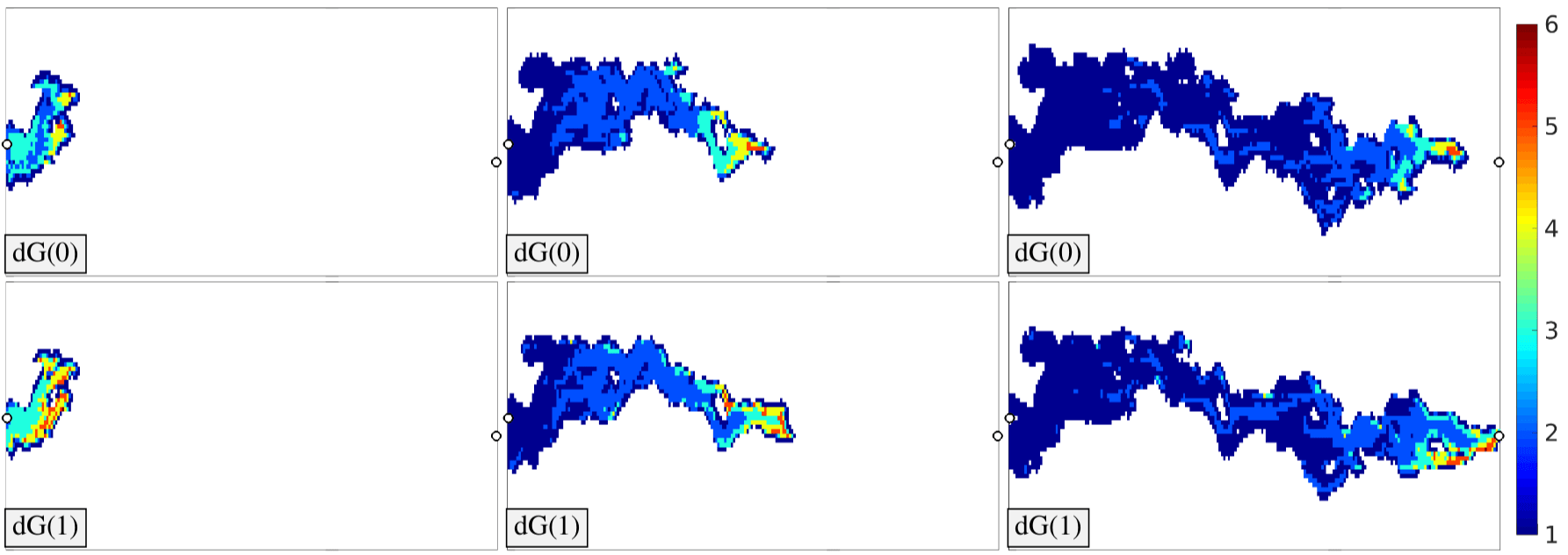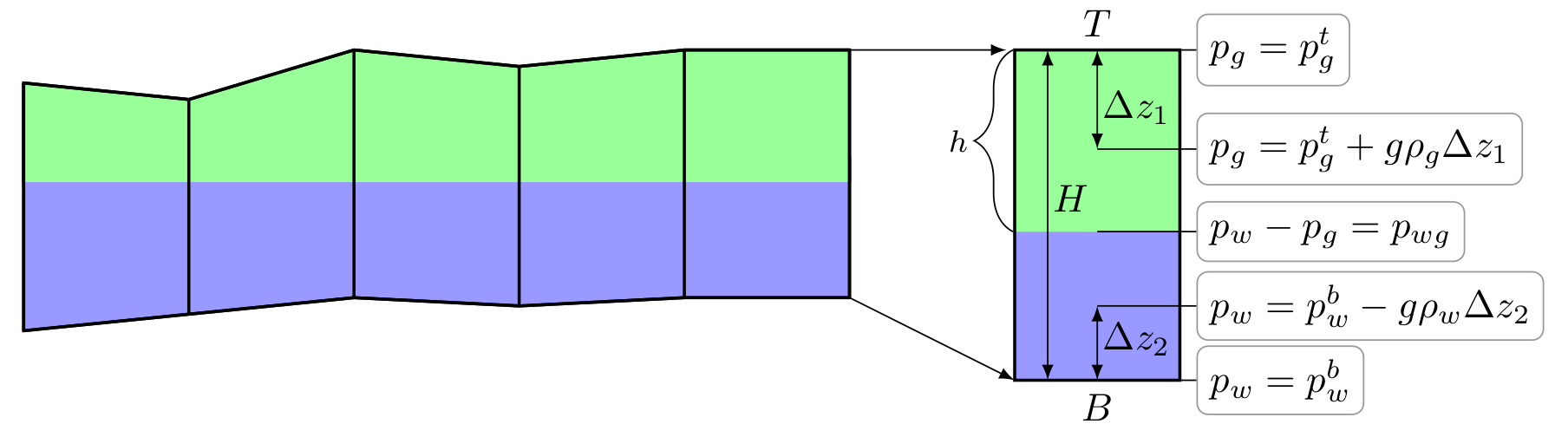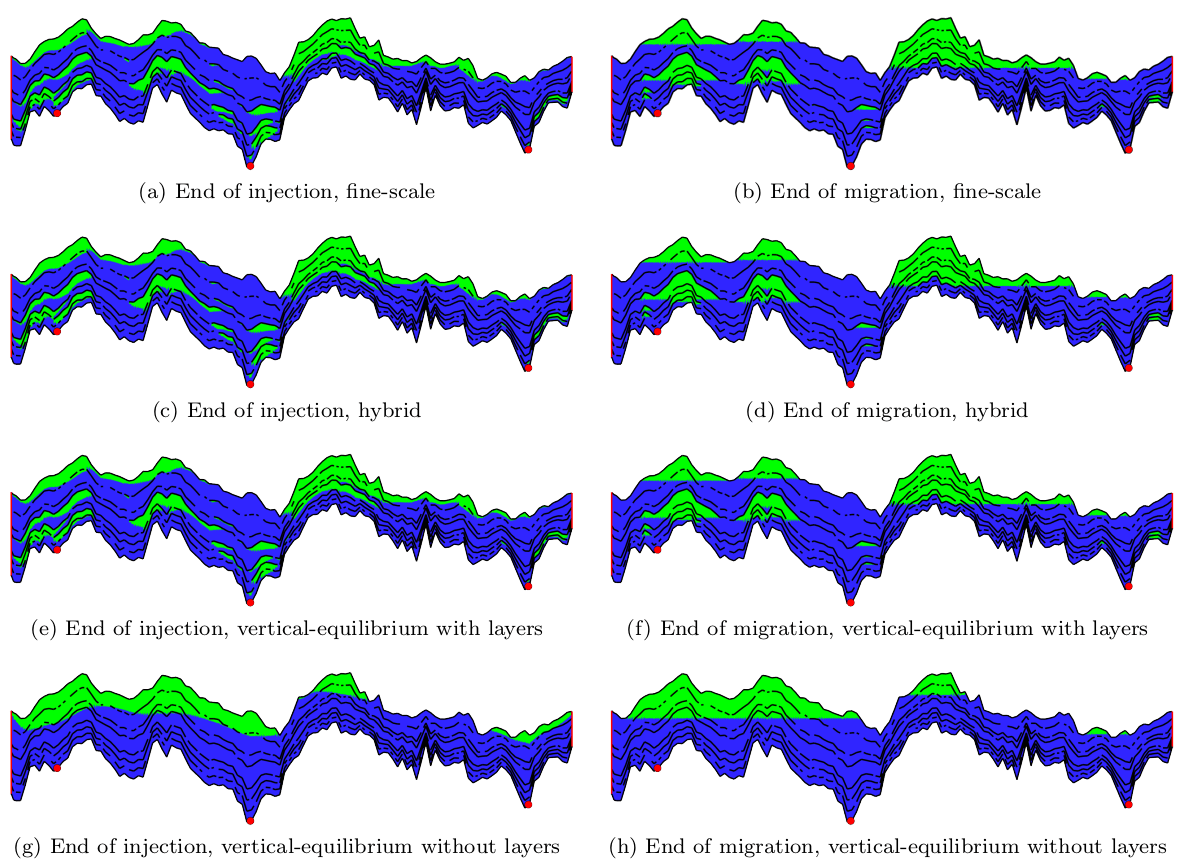# Using physics to speed up reservoir simulationBy Øystein Klemetsdal, PhD student at NTNU and SINTEF Digital, and Olav Møyner, Postdoc at NTNU and SINTEF Digital.

Reservoir simulation can in broad terms be described as simulation of fluids flowing in porous rock formations underground. Applications range from simulating the flow of oil and gas in petroleum reservoirs to determine optimal production strategies, to managing groundwater resources. In this article, we will not get down to bedrock of reservoir simulation (pun intended), but rather look into some of the challenges we face when simulating flow in porous media and discuss how knowledge of the underlying physics of the problem we are solving can be used to speed up simulations.

From a numerical point of view, flow in porous media poses many interesting challenges. Firstly, the PDEs describing subsurface flow are often strongly coupled and highly nonlinear. Secondly,  the computational grid must adapt to geological properties of the subsurface rock. In effect, a typically cell can span hundreds of meters in the lateral direction, but only a few meters in the vertical direction. Additionally, orders of magnitude variations in petrophysical properties and large variations in fluid velocities from stagnant and slow-moving flow regions to high-flow regions around wells impose severe CFL restrictions so that implicit discretizations are needed. Altogether, this requires robust and efficient nonlinear solution procedures to facilitate simulations on a field scale.

## Sequential splitting

On semi-discrete form with backward Euler temporal discretization, conservation of mass for a fluid phase$\alpha$ flowing in a porous medium can be written as$\tfrac{1}{\Delta t} \left( (\phi \rho_\alpha S_\alpha)^{n+1} - (\phi \rho_\alpha S_\alpha)^{n}\right) + \nabla \cdot \left(\rho_\alpha \vec v_\alpha \right) - q_\alpha = 0,$

where the macroscopic Darcy velocity$\vec v_\alpha$ is given by the multiphase extension of Darcy’s law:$\vec v_\alpha = -\frac{k_{r,\alpha}}{\mu_\alpha} K \nabla p_\alpha.$

The quantities$\phi$ and$K$ are properties of the subsurface rock: The porosity$\phi$ is the fraction of the rock that is void space, and the permeability tensor$K$ is a measure of the rock’s ability to transmit a fluid. In the multiphase extension of Darcy’s law, the permeability is augmented by the relative permeability$k_{r,\alpha}$. This, in turn, is a function of the fluid phase saturation$S_\alpha$, which is the fraction of the available pore volume occupied by phase$\alpha$. We require that all phases fill up the available pore volume, so that$\sum_\alpha S_\alpha = 1$. As usual,$\rho_\alpha$ and$\mu_\alpha$ denote the density and viscosity of phase$\alpha$, respectively, and$q_\alpha$ is a source or sink.

For cases with weak coupling between fluid pressure and saturation, we can obtain a significant reduction in computational costs by splitting the flow model into a pressure equation and a set of transport equations and solve them sequentially. We can do this by dividing the conservation equation above by$\rho_\alpha^{n+1}$ and summing the equations for all phases$\alpha$. Since the phases fill the pore volume ($\sum_\alpha S_\alpha = 1$), this eliminates$S_\alpha^{n+1}$ from the accumulation term. We term the resulting equation the  pressure equation, and the original conservation equations are termed transport equations. These equations tend to have a distinctly different mathematical character: the pressure equation is usually elliptic, whereas the transport equations tend to be hyperbolic.

In a sequential implicit solution procedure, we first solve for the pressure equation keeping saturations fixed. This gives us phase velocities$\vec v_\alpha$, which we can use to solve the transport equations, keeping the pressure fixed. The effect of using such a splitting scheme is two-fold: We solve two smaller subproblems instead of solving the full problem, and we can use highly specialized solvers to target each of the two subproblems.

## Reordering

We now introduce a grid$\{\Omega_i\}$ and assume for the sake of brevity that we have a discontinuous Galerkin (dG) discretization for our transport equations. By applying the divergence theorem, the flux term$\nabla \cdot \left(\rho_\alpha \vec v_\alpha \right)$ is commonly treated as a sum of integrals$\int \rho_\alpha\vec v_\alpha \cdot \vec n_{ij}$ over all faces of cell$\Omega_i$, where$\vec n_{ij}$ is the normal from cell$\Omega_i$ to cell$\Omega_j$ . In other words, the solution in cell$\Omega_i$ depends on the solution in its neighboring cells.

An important property of the equations above is that the flow is unidirectional along streamlines in the absence of gravity and capillary effects. If we evaluate quantities on the faces of cell$\Omega_i$ using an upstream approximation, we preserve this unidirectional property. This means that the solution in cell$\Omega_i$ only depends on the solution in cells that are upstream of$\Omega_i$, and is independent of all of its downstream cells.

Once we have solved the pressure equation, we can exploit this inherent locality by reordering our grid cells based on the intercell flux graph with cells as nodes and the fluxes$\vec v \cdot \vec n_{ij}$ as edges. This will be a directed, acyclic graph (DAG). Starting from an inflow source, we can then solve the transport equations in a very efficient manner cell-by-cell. The reordering technique is visualized below, where a fluid flows from a source in the lower left corner to a sink in the upper right corner. Here, the problem is discretized on a Voronoi grid using a dG(0) method. Notice how the discretization matrix becomes lower triangular after reordering.Sparsity pattern for a dG(0) method formulated on a Voronoi grid with original (left) and reordered (right) numbering of cells. The inset shows grid cell 35 and its upstream and downstream neighbors.

In practice we also have to deal with gravity and capillary forces, which means that the flow is no longer guaranteed to be unidirectional along streamlines. The resulting intercell flux graph will then contain cycles, and and at first glance, the neat reordering idea has apparently been debunked by something as trivial as gravity. Luckily, there is a way to deal with cycles: by grouping cells that are part of the same cycle into a single supernode, the resulting augmented graph is directed and acyclic. We can then traverse the graph and solve either single-cell problems, or small blocks of mutually dependent cells. The solution procedure can be summarized as follows:

1. Solve the pressure equation keeping saturation fixed to obtain intercell fluxes.
2. Construct a DAG based on the intercell flux graph by grouping cells that are part of the same cycle into a single supernode.
3. Starting from the first node in the DAG, solve for one cell or block of mutually dependent cells at the time.

Let us look at an example of how this reordering method works in practice. We extract a single layer of SPE10 Model 2, which is a widely used benchmark case in reservoir simulation. The petrophysical model is made up of a complex pattern of fluvial channels, and the injected fluid will finger rapidly through these. The reservoir is initially filled with a fluid of higher viscosity, e.g., oil, and we simulate injection of a fluid with lower viscosity, e.g., water. The layer is completely horizontal so that there are no gravity effects, and we neglect capillary pressure. In this case, the intercell flux graph will contain no cycles, so that we can solve the transport equations cell-by-cell. The resulting water saturation is shown at selected time steps below. Here, we have used both a first and second-order discontinuous Galerkin method.Evolving water front in a channeled reservoir using a first and second order discontinuous Galerkin method, labelled dG(0) and dG(1), respectively.

Below is the number of nonlinear iterations used to solve the single-cell problems at the same timesteps.Number of nonlinear iterations per cell at three instances in time corresponding to the solution profiles. No iterations were performed for cells in white.

We have only reported iterations for active cells. By active cells, we mean cells in which the water saturation was updated during the time step either because the residual at the beginning of the time step was above the nonlinear tolerance, or because one or more of the cell’s upstream neighbors were updated during the time step. The inactive cells are coloured white, since the solution of that timestep did not require an update in these cells. We see that active cells amount to a small fraction of the total number of cells, emphasizing a major advantage of the reordering method: Most of the flow is located to the channels, and there is very little happening elsewhere. By using reordering, we avoid solving for a bunch of zeros and instead focus our computational resources on the part of the domain where updates are nonzero.

Another attractive feature of this method is its effect on the nonlinear solution procedure: Whereas solving for all cells simultaneously requires iterating until the residual in the most problematic cell has converged, a cell-by-cell solution procedure means that all cells can be solved using only their required minimum number of iterations. Finally, the significant speedup of the reordering method facilitates the use of a higher-order spatial discretization method, as long as this preserves the unidirectional dependence of the flow equations. Using higher-order methods for realistic industrial-scale reservoirs would otherwise tend to be prohibitively expensive. Higher-order methods are for instance beneficial when simulating enhanced oil recovery scenarios, whose results otherwise tend to be masked by numerical diffusion.

See Klemetsdal et. al, Nonlinear Gauss-Seidel Solvers With Higher Order For Black-Oil Models, ECMOR XVI – 16th European Conference on the Mathematics of Oil Recovery, 2018.

## Vertical equilibrium models

Another application of a similar set of governing equations is the simulation of geological CO$_2$ sequestration in saline aquifers. The CO$_2$ may originate from a number of different sources: It may be separated from the production of CO$_2$-rich gas fields, captured from the combustion process in power plants, from cement production, or any number of other industrial processes that emit CO$_2$.

Simulating how CO$_2$ migrates through a saline aquifer is especially challenging due to large variations of in temporal and spatial scales. Whereas gravity segregation between the heavy resident brine in the aquifer and the lighter supercritical CO$_2$ phase takes place over as little as hours or minutes, permanent long-term storage in terms of mineralization of CO$_2$ through a pathway of dissolution can take thousands of years to achieve. Aquifers may have a lateral length of tens of thousands of kilometers, while the CO$_2$ plume itself typically is a few decimeters or meters thin and thus requires an infeasible number of grid cells to be resolved with sufficient vertical accuracy.

One proposed approach for simulating CO$_2$ storage is a revival of older techniques from the early days of numerical simulation, when a full 3D representation for reservoir simulation was difficult to achieve with the computers of the day. Under the assumption of rapid gravity segregation between the light CO$_2$ phase and the heavier brine, the equations can be reformulated in a pseudo-3D form by integrating the flow equations in the vertical direction (and possibly assuming that vertical equilibrium is reached instantaneously).  This not only reduces the number of grid cells significantly, but also reduces the span in time constants, thereby allowing for significantly faster simulation of how the CO$_2$ plume spreads laterally.

The distribution of pressure and CO$_2$ saturation, as shown below, can then be reconstructed analytically from the assumption of hydrostatic equilibrium in the vertical direction.Pressure reconstruction from the assumption of hydrostatic vertical equilibrium.

One limit of this approach is that the assumption of complete segregation in the vertical direction may not be valid, especially in the presence of impermeable layers or near injection sites. A recent paper from NTNU and SINTEF describes how to systematically partition the flow compartments vertically and laterally and then couple the different discretization regions, allowing for more accurate simulation results in a fraction of the time it takes to simulate a full model. In other words, scenarios that would take hours or days to evaluate on a workstation can be simulated on a regular laptop in the time it took you to read this blog post.A cross-section of the Utsira aquifer with a synthetic injection scenario and a stochastic distribution of impermeable layers. The CO2 is plotted in green, and flows to the top of each flow compartment. The layered vertical equilibrium model is approximately 165 times faster to simulate than the original fine-scale model, with no apparent loss in accuracy.

See Møyner & Nilsen, Multiresolution coupled vertical equilibrium model for fast ﬂexible simulation of CO2 storage, Computational Geosciences, 2018

## Closing remarks

This article highlights some of the benefits of incorporating physical knowledge into numerical solution procedures. The result is a significant speedup without significant loss of accuracy. In the industry, reservoir simulation is often used to quantify uncertainty or optimize production strategies. This typically involves simulating thousands of plausible realizations of the same reservoir with slightly different parameter settings. The need for a fast simulator is then obvious. In addition, a fast simulator may also facilitate simulations that would be too expensive using conventional simulation techniques, such as simulation of long-term CO2-storage in aquifer-scale systems and high-resolution simulation of enhanced oil recovery.